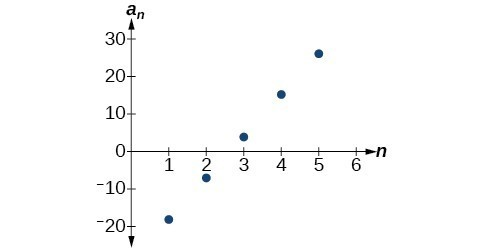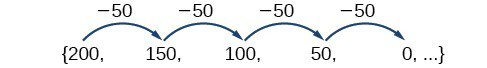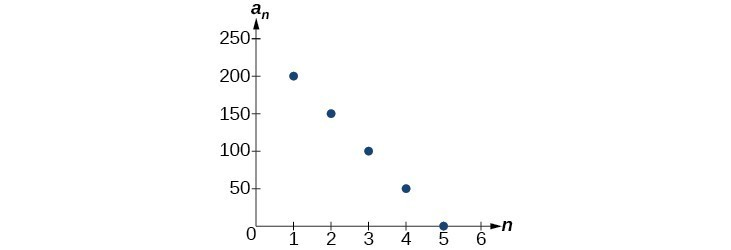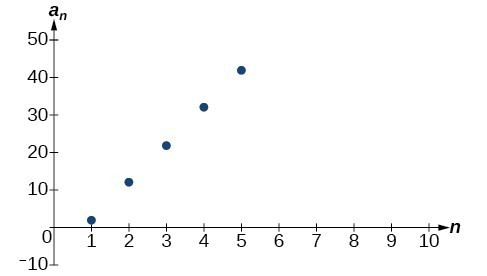## Using Formulas for Arithmetic Sequences

Some arithmetic sequences are defined in terms of the previous term using a recursive formula. The formula provides an algebraic rule for determining the terms of the sequence. A recursive formula allows us to find any term of an arithmetic sequence using a function of the preceding term. Each term is the sum of the previous term and the common difference. For example, if the common difference is 5, then each term is the previous term plus 5. As with any recursive formula, the first term must be given.

$\begin{array}{lllll}{a}_{n}={a}_{n - 1}+d\hfill & \hfill & \hfill & \hfill & n\ge 2\hfill \end{array}$

### A General Note: Recursive Formula for an Arithmetic Sequence

The recursive formula for an arithmetic sequence with common difference $d$ is:

$\begin{array}{lllll}{a}_{n}={a}_{n - 1}+d\hfill & \hfill & \hfill & \hfill & n\ge 2\hfill \end{array}$

### How To: Given an arithmetic sequence, write its recursive formula.

1. Subtract any term from the subsequent term to find the common difference.
2. State the initial term and substitute the common difference into the recursive formula for arithmetic sequences.

### Example 4: Writing a Recursive Formula for an Arithmetic Sequence

Write a recursive formula for the arithmetic sequence.

$\left\{-18,-7, 4, 15, 26,\dots \right\}$

### Solution

The first term is given as $-18$ . The common difference can be found by subtracting the first term from the second term.

$d=-7-\left(-18\right)=11$

Substitute the initial term and the common difference into the recursive formula for arithmetic sequences.

$\begin{array}{l}{a}_{1}=-18\hfill \\ {a}_{n}={a}_{n - 1}+11,\text{ for }n\ge 2\hfill \end{array}$

### Analysis of the Solution

We see that the common difference is the slope of the line formed when we graph the terms of the sequence, as shown in Figure 3. The growth pattern of the sequence shows the constant difference of 11 units.Figure 3

### How To: Do we have to subtract the first term from the second term to find the common difference?

No. We can subtract any term in the sequence from the subsequent term. It is, however, most common to subtract the first term from the second term because it is often the easiest method of finding the common difference.

### Try It 5

Write a recursive formula for the arithmetic sequence.

$\left\{25,37,49,61,\dots \right\}$

## Using Explicit Formulas for Arithmetic Sequences

We can think of an arithmetic sequence as a function on the domain of the natural numbers; it is a linear function because it has a constant rate of change. The common difference is the constant rate of change, or the slope of the function. We can construct the linear function if we know the slope and the vertical intercept.

${a}_{n}={a}_{1}+d\left(n - 1\right)$

To find the y-intercept of the function, we can subtract the common difference from the first term of the sequence. Consider the following sequence.The common difference is $-50$ , so the sequence represents a linear function with a slope of $-50$ . To find the $y$ -intercept, we subtract $-50$ from $200:200-\left(-50\right)=200+50=250$ . You can also find the $y$ -intercept by graphing the function and determining where a line that connects the points would intersect the vertical axis. The graph is shown in Figure 4.Figure 4

Recall the slope-intercept form of a line is $y=mx+b$. When dealing with sequences, we use ${a}_{n}$ in place of $y$ and $n$ in place of $x$. If we know the slope and vertical intercept of the function, we can substitute them for $m$ and $b$ in the slope-intercept form of a line. Substituting $-50$ for the slope and $250$ for the vertical intercept, we get the following equation:

${a}_{n}=-50n+250$

We do not need to find the vertical intercept to write an explicit formula for an arithmetic sequence. Another explicit formula for this sequence is ${a}_{n}=200 - 50\left(n - 1\right)$ , which simplifies to ${a}_{n}=-50n+250$.

### A General Note: Explicit Formula for an Arithmetic Sequence

An explicit formula for the $n\text{th}$ term of an arithmetic sequence is given by

${a}_{n}={a}_{1}+d\left(n - 1\right)$

### How To: Given the first several terms for an arithmetic sequence, write an explicit formula.

1. Find the common difference, ${a}_{2}-{a}_{1}$.
2. Substitute the common difference and the first term into ${a}_{n}={a}_{1}+d\left(n - 1\right)$.

### Example 5: Writing the nth Term Explicit Formula for an Arithmetic Sequence

Write an explicit formula for the arithmetic sequence.

$\left\{2, 12, 22, 32, 42, \dots \right\}$

### Solution

The common difference can be found by subtracting the first term from the second term.

$\begin{array}{ll}d\hfill & ={a}_{2}-{a}_{1}\hfill \\ \hfill & =12 - 2\hfill \\ \hfill & =10\hfill \end{array}$

The common difference is 10. Substitute the common difference and the first term of the sequence into the formula and simplify.

$\begin{array}{l}{a}_{n}=2+10\left(n - 1\right)\hfill \\ {a}_{n}=10n - 8\hfill \end{array}$

### Analysis of the Solution

The graph of this sequence, represented in Figure 5, shows a slope of 10 and a vertical intercept of $-8$ .Figure 5

### Try It 6

Write an explicit formula for the following arithmetic sequence.

$\left\{50,47,44,41,\dots \right\}$